Communication, Amplitude Modulation & Demodulation

# Communication, Amplitude Modulation & Demodulation - Notes | Study Communication System - Electronics and Communication Engineering (ECE)

 Table of contentsCOMMUNICATION:Block diagram of a communication system:Modulation:Amplitude Modulation:Frequency Spectrum of AM:The concept of Negative Frequency:Modulation Index:Power Relation in AM Wave:Current Relations in AM Wave:Voltage Relations in AM Wave:Modulated by Several Sine Waves:Transmission Efficiency:Generation of AM:Square Law Modulator:Demodulation of AM:Square Law Detector:Envelope Detector:1 Crore+ students have signed up on EduRev. Have you?

COMMUNICATION:

• Communication is a process by which information is exchanged between individuals through a common system of symbols
• It is a technique for expressing ideas effectively
• It is a system of routes for moving troops, supplies, and vehicles
• Communication is the transfer of information from one point in space and time to another point.

Block diagram of a communication system: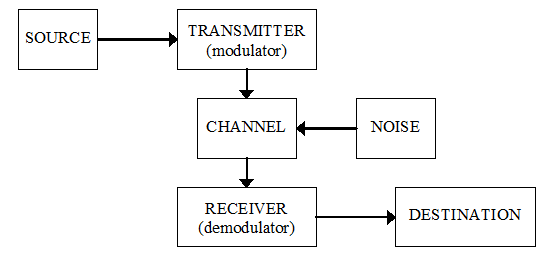Transmitter: couples the message onto the channel using high-frequency signals

Receiver:  restores the signal to its original form

Channel: the medium used for transmission of signals

Modulation: the process of shifting the frequency spectrum of a message signal to a frequency range in which more efficient transmission can be achieved

Demodulation: the process of shifting the frequency spectrum back to the original baseband frequency range and reconstructing the original form, if necessary

Modulation:

• Modulation may be defined as the process by which some characteristics of a signal called carrier is varied in accordance with the instantaneous value of another signal called modulating the signal.
• The carrier frequency is greater than the modulating frequency. The signal resulting from the process of modulation is called the modulating signal.
• Types of Modulation: When the carrier wave is continuous in nature, the modulation process is known as Continuous Wave (CW) modulation or analog modulation.

1. Amplitude Modulation

2. Angle Modulation

Amplitude Modulation:

• A system of modulation in which the envelope of the transmitted wave contains a component similar to the waveform of the signal to be transmitted.
• The envelope of the modulated carrier has the same shape as the message waveform, achieved by adding the translated message that is appropriately proportional to the unmodulated carrier.
• Amplitude modulation may be defined as a system in which the maximum amplitude of the carrier wave is made proportional to the instantaneous value (amplitude) of the modulating or baseband signal.
• Let c(t) = Vc cos ωct, and m(t) = Vm sin ωmt. Then the amplitude modulated signal is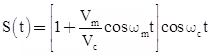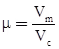where µ is known as modulation index.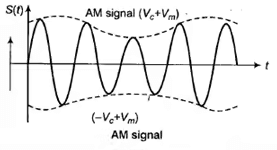Frequency Spectrum of AM:

• Significant frequencies from fc to (fc + fm) is called an upper sideband.
• Significant frequencies from (fc - fm) to fc is called as lower sideband.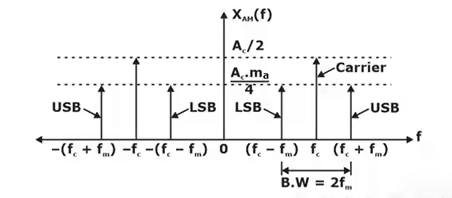fc = Carrier frequency fm = Modulating signal frequency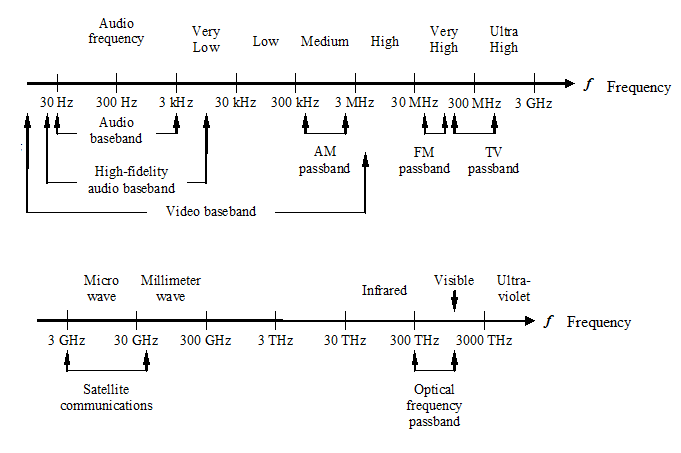• A signal can be transmitted in different frequency bands.
• If the signal is transmitted over its original frequency band, the transmission is called baseband transmission.
• If the signal is shifted to a frequency band higher than its original baseband, it is called passband transmission.

The concept of Negative Frequency:

The negative frequency appeared in a spectrum is due to the exponential representation of Fourier transform. It is helpful to consider only the positive frequency region while treating the negative frequency region as a replica of the positive frequencies. AM signal bandwidth is BW = (fc + fm) - (fc - fm) = 2fm The bandwidth of the amplitude modulated was is twice the highest frequency present in the baseband or modulating signal.

Carrier signal [i.e., c(t) = Vccos 2πfct] is a fixed frequency signal having frequency ωc.

The modulating or baseband signal x(t) contains the information or intelligence to be transmitted.

In the process of amplitude modulation, the frequency and phase of the carrier remain constant whereas the maximum amplitude varies according to the instantaneous value of the information signal.

The AM wave has a time-varying amplitude called as the envelope of the AM wave.

We generally keep fc ≥ fm, which ensures that the two sidebands do not overlap each other.

Modulation Index:

• In AM system, the modulation index is defined as the measure of the extent of amplitude variation about an unmodulated maximum carrier.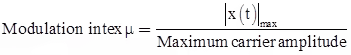or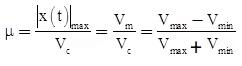• Vmax is a Maximum value of amplitude modulated wave, and Vmin is a Minimum value of amplitude modulated wave.
• The baseband or modulating signal will be preserved in the envelope of the AM signal only if we have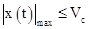• The modulation index is less than or equal to unity.
• If μ > 1or the percentage modulation is greater than 100, the baseband signal is not preserved in the envelope.
• Minimum value of amplitude modulated wave is Vmin = (Vc - Vm)
• Maximum value of amplitude modulated wave is Vmax = (Vc + Vm)
• For avoiding phase reversal | μ | < 1

Power Relation in AM Wave:

The total power PAM of the AM wave is the sum of the carrier power Pc and sideband power Ps.

PAM = Pc + Ps

PAM=PC+PLSB+PUSB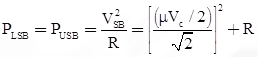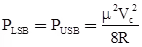• where, PLSB = Lower sideband power, PUSB = Upper sideband power, and Pc = Carrier signal power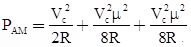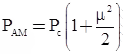• Maximum power dissipated in the AM wave is PAM = 1.5 Pc for μ = 1 and this is maximum power that amplifier can handle without distortion.

Current Relations in AM Wave:

• The total power and carrier power can be represented by the following equations: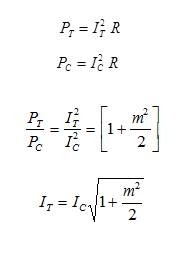• IC is the unmodulated carrier current and IT  is the total, or modulated, the current of an AM transmitter.
• These currents are usually applied or measured at the antenna. Hence, R is the antenna resistance.

Voltage Relations in AM Wave:

• The total modulating voltage in AM wave  in terms of carrier voltage can be given as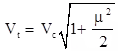• where, Vt = Total modulating voltage, and Vc = Carrier voltage

Modulated by Several Sine Waves:

• Let V1, V2, V3...... etc be the simultaneous modulation voltages. Then the total modulating voltage Vt will be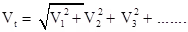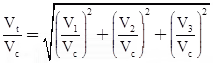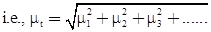• where μt is overall modulation index, μ1, μ2, μ3 are respective modulation index for individual waves.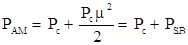• where PSB is total sideband power.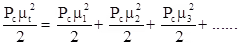Transmission Efficiency:

• In AM wave, transmission efficiency may be defined as the percentage of total power contributed by the sidebands.
• Transmission efficiency,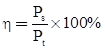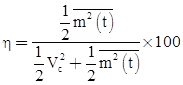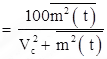• The maximum transmission efficiency of the AM is only 33.33%. This implies that only one-third of the total power is carried by the sidebands and the rest two-thirds is wasted.
• In AM, it is generally more convenient to measure the AM transmitter current than the power.
• The modulation index may be calculated from the values of unmodulated and modulated currents in the AM transmitter.

Generation of AM:

• The device which is used to generate an amplitude modulated (AM) wave is known as the amplitude modulator.
• The methods of AM generation may be broadly classified as following

1. Low-level AM modulation

2. High-level AM modulation

Square Law Modulator:

• Square law diode modulation and switching modulation are examples of low-level modulation because it is suited at low voltage levels (i.e., because of non-linear V-I characteristics of diode)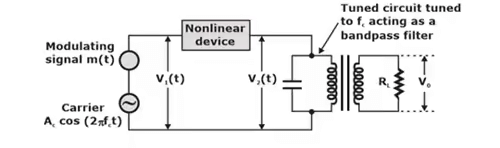Square Law Modulator

• The non-linear relationship between voltage and current for a diode is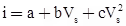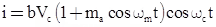• Modulation index (μ or ma)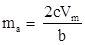Demodulation of AM:

• The process of extracting a modulating or baseband signal from the modulated signal is called demodulation or detection.
• For amplitude modulation, detectors or demodulators are categorised as
• Square law detectors
• Envelope detectors

Square Law Detector:

• A low-level amplitude modulated signal can only be detected by using square law detectors in which a device operating in the non-linear region is used to detect the modulating signal.
• It usually detects the signal of below 1 volt.
• The current equation is :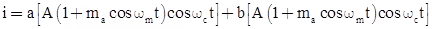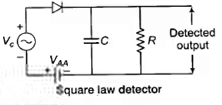Envelope Detector:

• AM signal with a large carrier is detected by using the envelope detector.
• The envelope detector uses the circuit which extracts the envelope of the AM wave.
• A diode operating in a linear region of its V-I characteristics can extract the envelope of an AM wave. This type of detector is known as envelope detector or a linear detector.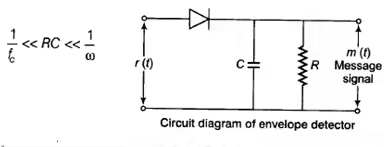• If the time constant RC is quite low, which results in large fluctuations in an output voltage.
• Whereas, if the time constant RC is very large, then misses several peaks of the rectified output voltage during negative peaks.
• If RC is too low, the output voltage waveform is spiky in nature. On the other hand, if RC is too large, distortion may result due to clipping during the negative peaks of the modulation wave.
The document Communication, Amplitude Modulation & Demodulation - Notes | Study Communication System - Electronics and Communication Engineering (ECE) is a part of the Electronics and Communication Engineering (ECE) Course Communication System.
All you need of Electronics and Communication Engineering (ECE) at this link: Electronics and Communication Engineering (ECE)

37 docs|22 tests

## Communication System

37 docs|22 tests

Track your progress, build streaks, highlight & save important lessons and more!

,

,

,

,

,

,

,

,

,

,

,

,

,

,

,

,

,

,

,

,

,

,

,

,

;